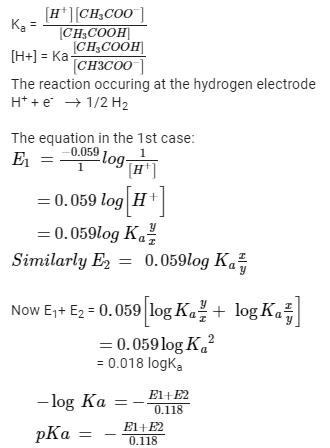Courses

# A hydrogen electrode placed in a buffer solution of CH3COONa and acetic acid in the ratio’s x : y and y : x has electrode potential values E1 volts and E2 volts respectively at 25°C. The pKa values of acetic acid is (E1 and E2 are oxidation potential)a)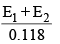b)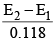c)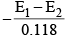d)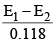Correct answer is option 'A'. Can you explain this answer? Related Test: Test: Electrochemistry- 2

## IIT JAM Question

By Ajit Yadav · 2 weeks ago ·IIT JAM
Divya Gaur answered Nov 12, 2018
CH3COOH → CH3COO^- + H^+
H2O → H^+ + OH^-